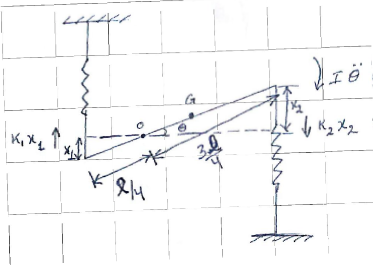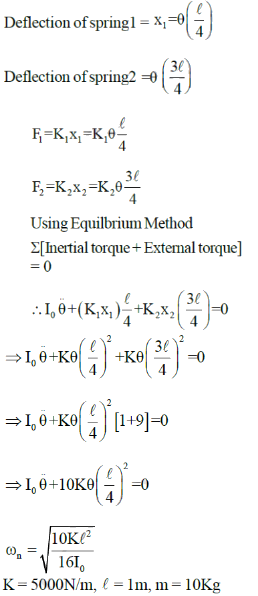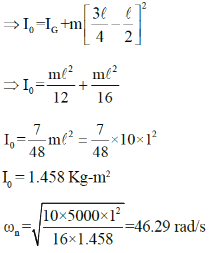Courses

# Mechanical Vibrations

## 25 Questions MCQ Test GATE Mechanical (ME) 2022 Mock Test Series | Mechanical Vibrations

Description
This mock test of Mechanical Vibrations for GATE helps you for every GATE entrance exam. This contains 25 Multiple Choice Questions for GATE Mechanical Vibrations (mcq) to study with solutions a complete question bank. The solved questions answers in this Mechanical Vibrations quiz give you a good mix of easy questions and tough questions. GATE students definitely take this Mechanical Vibrations exercise for a better result in the exam. You can find other Mechanical Vibrations extra questions, long questions & short questions for GATE on EduRev as well by searching above.
QUESTION: 1

Solution:
QUESTION: 2

### It is desired to make a product having T-shaped cross-section from a rectangular aluminium block. Which one of the following processes is expected to provide the highest strength of the product?

Solution:
*Answer can only contain numeric values
QUESTION: 3

### An instrument has a natural frequency of 10 Hz. Maximum acceleration of the system is observed to be 24 m/s2. The maximum displacement of the system is ....mm. (Important - Enter only the numerical value in the answer)

Solution: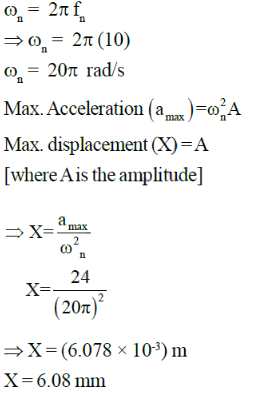QUESTION: 4

The displacement of particle undergoing S.H.M. follows the equation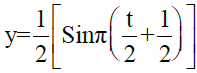where y is displacement in cm and t is the time. The maximum acceleration of the particle is approximately.

Solution: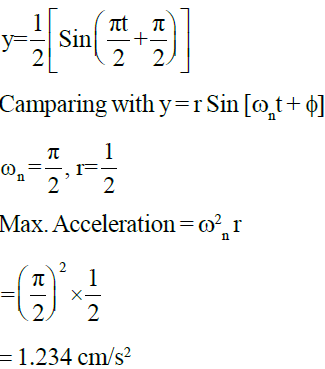*Answer can only contain numeric values
QUESTION: 5

Common Data Question 5 & 6

An underdamped shock absorber is to be designed for a motor cycle of mass 200Kg such that during a road
bump, the damped period of vibration is limited to 2sec and the amplitude of vibration should recduce to one sixteenth in one cycle.

Q. The stiffness of the spring used is......KN/m

(Important - Enter only the numerical value in the answer)

Solution:

Mass of motor cycle m = 200Kg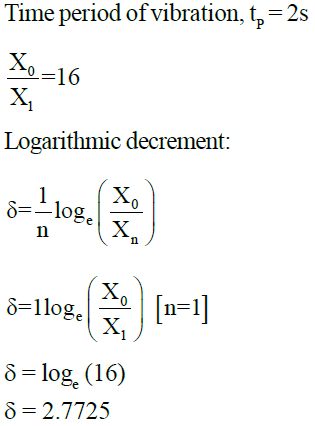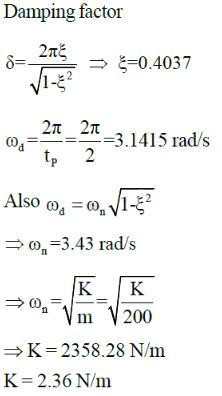*Answer can only contain numeric values
QUESTION: 6

Common Data Question 5 & 6

An underdamped shock absorber is to be designed for a motor cycle of mass 200Kg such that during a road
bump, the damped period of vibration is limited to 2sec and the amplitude of vibration should reduce to one sixteenth in one cycle.

Q. The damping coefficient of the shock absorber is ...... N-s/m

(Important - Enter only the numerical value in the answer)

Solution:

Damping coefficient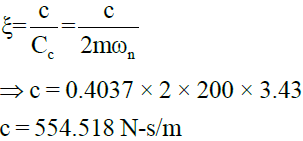*Answer can only contain numeric values
QUESTION: 7

In a vibrating system a mass of 3 Kg is suspended by a spring of stiffness 1200 N/m and it is subjected to a harmonic exicitation of 20N. If the viscous damper is provided with the damping coefficient of 75 N-s/m, the
amplitude at resonance is...m

(Important - Enter only the numerical value in the answer)

Solution: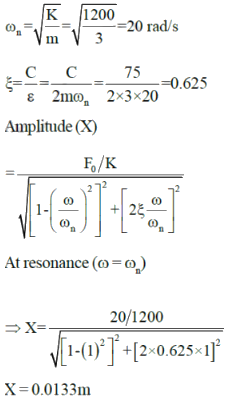*Answer can only contain numeric values
QUESTION: 8

Common data Questions 8 & 9
In a forced vibratory system a body having 2Kg mass vibrates in a viscous fluid. The harmonic excitation force of 20N acting on the mass results in a resonance amplitude of 15mm with a period of 0.15 sec.

The damping coefficient of viscous fluid is....N-s/m

(Important - Enter only the numerical value in the answer)

Solution: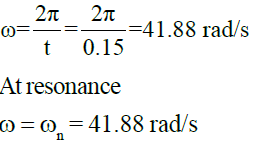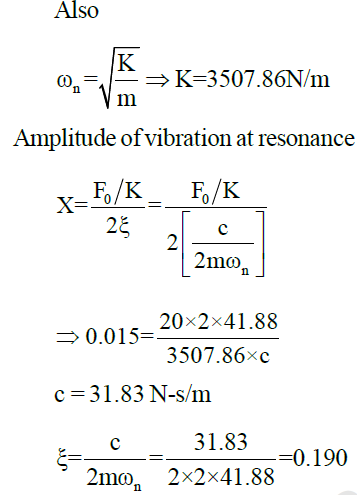*Answer can only contain numeric values
QUESTION: 9

If the system is excited by the same harmonic force but at a frequency of 5 cycles/s. The amplitude of
forced vibrations with damper is.......m

(Important - Enter only the numerical value in the answer)

Solution: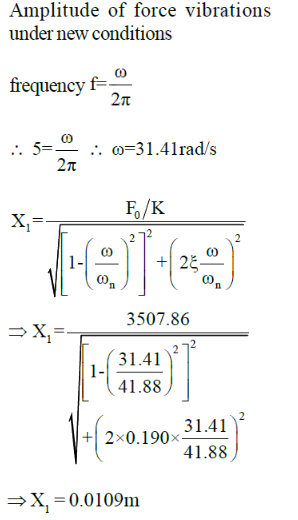*Answer can only contain numeric values
QUESTION: 10

A vertical single stage compressor has a mass of 500 Kg mounted on springs having stiffness of 1.96 × 105 N/m and a dashpot having damping factor of 0.2. The rotating parts are completely balanced and the equivalent reciprocating parts weigh 2 Kg. The stroke of the compressor is 0.2m. The dynamic amplitude of vertical motion is.....mm
[Take operating speed = 200 rpm]

(Important - Enter only the numerical value in the answer)

Solution: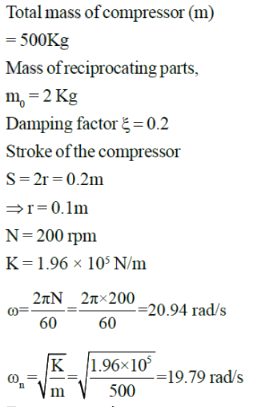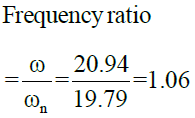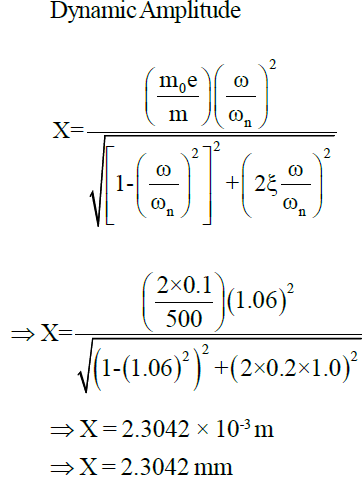*Answer can only contain numeric values
QUESTION: 11

An automobile is found to have a natural frequency of 20 rad/s without load and 17.32 rad/s with load of mass 500 kg. The mass of the automobile by treating it as single degree of freedom is......Kg

(Important - Enter only the numerical value in the answer)

Solution: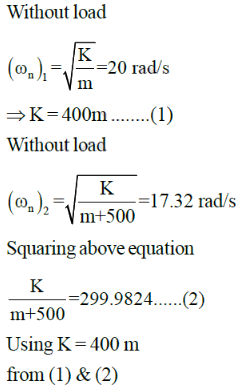m = 1499.6481 Kg

QUESTION: 12

A spring mass system with mass 2 Kg and stiffness 3200 N/m has an initial displacement of x0 = 0. The
maximum initial velocity that can be given to the mass without the amplitude of free vibration exceeding a value of 0.1m is

Solution: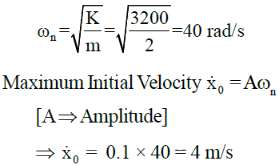*Answer can only contain numeric values
QUESTION: 13

A vibrating system consists of mass = 14 Kg, stiffness of spring = 1000 N/m and damping coefficient of dashpot = 25 N-s/m. The ratio of the amplitude after 6th cycle to the amplitude after 10th cycle is....

(Important - Enter only the numerical value in the answer)

Solution: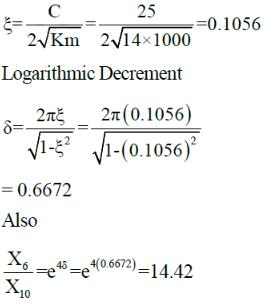*Answer can only contain numeric values
QUESTION: 14

A spring mass system with m = 10 Kg and K = 5000 N/m is subjected to a harmonic force of amplitude
250N and frequency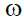. If the maximum amplitude of the mass is observed to be 100mm, the value ofis.....rad/s

(Important - Enter only the numerical value in the answer)

Solution: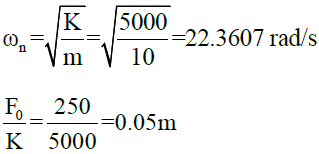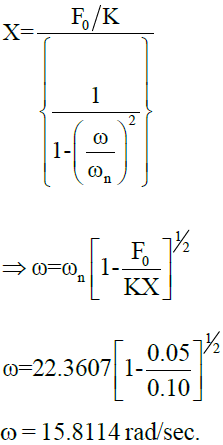QUESTION: 15

A mass m is suspended from a spring of stiffness 4000 N/m and is subjected to a harmonic force having an amplitude of 100N and a frequency of 5Hz. The amplitude of the forced motion of mass is observed to be 20mm. The value of m is nearly

Solution: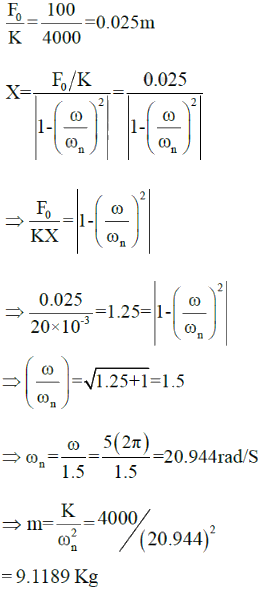QUESTION: 16

Which of the following statements is/are false for pneumatic isolators ?

1. Pneumatic isolators are used when driving frequencies below 10 Hz are present
2. Nausea is caused due to noise at low frequencies
3. At high natural frequencies, isolators can obtain efficiency of 90%
4. Pneumatic isolators have limited load carrying capacity

Solution:
QUESTION: 17

A wooden rectagular prism of density (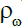) , height (h) and cross-section (a × b) is initialy dipped in an oil tub upto height x (h > x) and made to vibrate freely in vertical direction. The natural frequency of the vibration of the prism is observed to be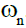. Now if the prism is replaced by a uniform circular cylinder of radius (r), height (h) and density (), The natural frequency of the vibration of the circular cylinder will be [Desity of oil is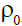]

Solution: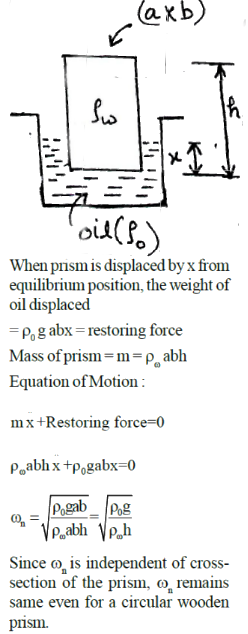*Answer can only contain numeric values
QUESTION: 18

The ratio of successive amplitudes of a viscously damped single degree of freedom system is found to be
[18:1]. The ratio of the succesive amplitudes if the amount of damping is doubled will be

(Important - Enter only the numerical value in the answer)

Solution: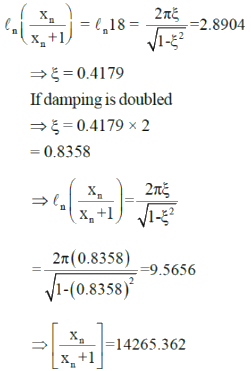*Answer can only contain numeric values
QUESTION: 19

For a spring mass damper system, m = 50Kg and K = 5,000 N/m. The damped natural frequency when C=
Cc/2 is.......rad/s

(Important - Enter only the numerical value in the answer)

Solution: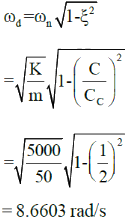QUESTION: 20

The natural frequency (in rad/s) of vibration of the given system is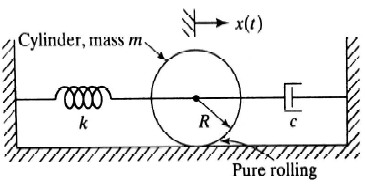Solution: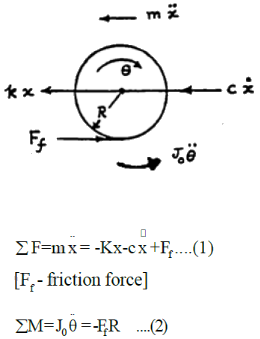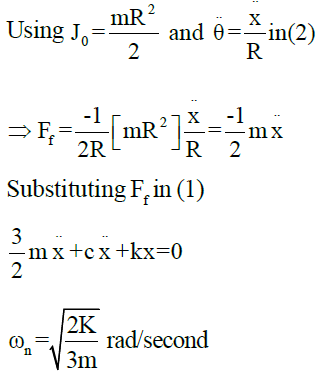QUESTION: 21

The circular disc of mass m and radius r is pivoted at point O as shown. If the disc is rotated by an angle ‘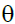’ and is left to vibrate, the natural frequency [in rad/sec] of the vibration of the system is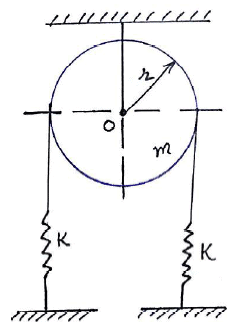Solution:

Let the disc be rotated by an anglein clock wise direction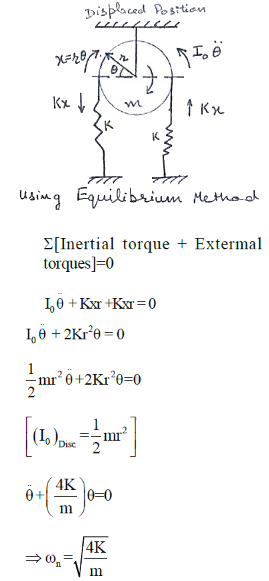QUESTION: 22

The natural frequency [in rad/s] of vibration of spring mass system shown in the figure is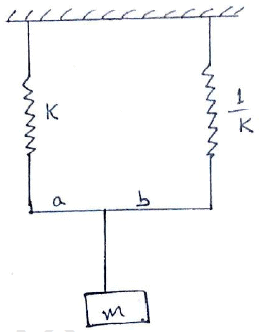Solution: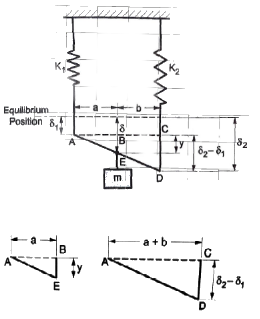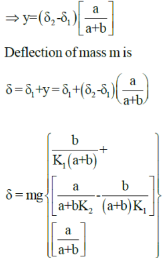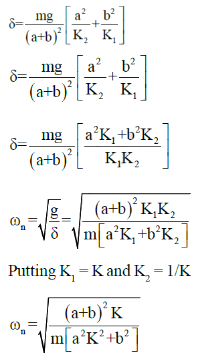*Answer can only contain numeric values
QUESTION: 23

A centrifugal pump, weighing 600N and operating at 1000 rpm, is mounted on six springs of stiffness 6000 N/m each. The maximum permissible unbalnce in order to limit the steady state deflection to 5mm peak to peak is...N-m

(Important - Enter only the numerical value in the answer)

Solution: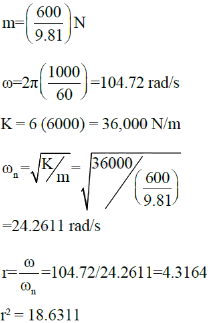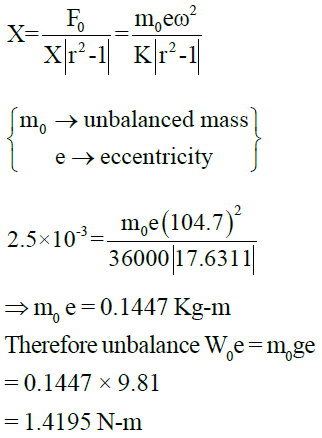QUESTION: 24

The reduction of the amplitude ratio in the presence of damping is very significant

Solution:
*Answer can only contain numeric values
QUESTION: 25

The natural frequency (in rad/s) of the given system is.....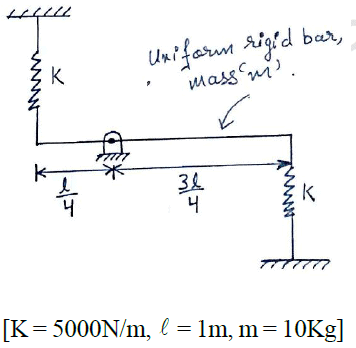Solution:

Let the rod be rotated about the hinge by a small angle